# Pre-Algebra : Commutative Property of Multiplication

## Example Questions

### Example Question #1 : Identities And Properties

Which property is illustrated by the example: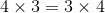Distributive property

Multiplicative identity property

Associative property of multiplication

Commutative property of multiplication

Commutative property of multiplication

Explanation:

The commutative property of mulitplication states that the order in which 2 numbers are multiplied does not affect the result: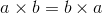Or in this case,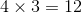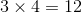### Example Question #2 : Commutative Property Of Multiplication

Use the Commutative Property of Multiplication to write the below expression in a different way.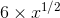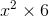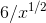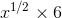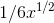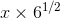Explanation:

The rule for Commutative Property of Multiplication isUsing this rule, the expressioncan be written as### Example Question #3 : Commutative Property Of Multiplication

Which of the following equations correctly shows the commutative property of multiplication?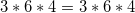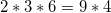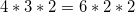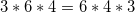Explanation:

The commutative property states that factors can be multiplied in any order and the product is always the same. While each of our answer choices are real equations, only one correctly displays this property: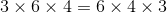### Example Question #4 : Commutative Property Of Multiplication

Evaluate the following expression without using a calculator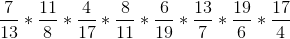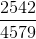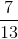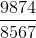Explanation:

This problem could easily turn into some super heavy calculations, but nobody has time for that.  Instead, we can make this problem very easy by utilizing the commutative property of multiplication, which in essence says that numbers can be multiplied in any order.

In other words,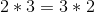and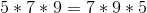That means that rather multiplying our numbers in their orginal order, which can actually change the order of the numbers without affecting the final product.  At this point we should notice that one of our numbers iswhile another is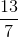.  Similarly the fractions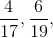and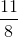are each accompanied by their recripocals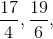and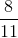respecitively.  At this point we must also remember the multiplicative inverse property, which tells us that the product of a number and its reciprocal will always be 1.  With that in mind, the strategic way to order the multiplication is as follows: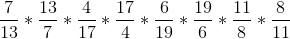Doing this pairs each number up with its reciprocal.  Finally, we can utlize the associative properpty of multiplication, which tells us that we can multiply any two numbers at a time.

In other words,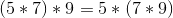.  This allows us to pair off the multiplication as follows.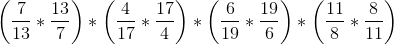Within each parentheses we now have a number multiplied by its reciprocal.  Remembering that this always equals 1, we can simplify, giving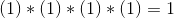### Example Question #5 : Commutative Property Of Multiplication

Which answer shows the communitive property of multiplication to the equation below?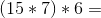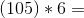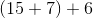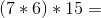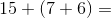Explanation:

The communitive property of multiplication is a property that states that the numbers can be multiplied in any combination. To show which answer is correct simply regroup the numbers in a different or and you will have your answer.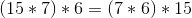### Example Question #6 : Commutative Property Of Multiplication

Which of the following displays the commutative property of multiplication?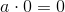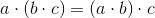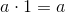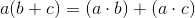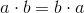Explanation:

The commutative property of multiplication states that 2 numbers can be multiplied in any order. Therefore,displays the commutative property of multiplication.

### All Pre-Algebra Resources